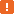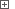# Obtaining Selected Rows in Developer Express QuantumGridWhen working with QuantumGrid controls, you may need to simulate the selection of several grid rows. This topic explains how to obtain values from the selected rows.In order for TestComplete to be able to perform these actions, the following conditions must be met: The tested application must be compiled with debug information included (see Preparing Application With QuantumGrid Controls for TestComplete). The Delphi and C++Builder Application Support and Developer Express Control Support plugins must be installed and enabled in TestComplete. The former plugin make Delphi and C++Builder applications Open to TestComplete. The latter plugin adds the `DevExpressQuantumGrid` object that provides support for QuantumGrid (TcxGrid) controls; without it you will not be able to work with QuantumGrid controls using their internal methods and properties. Note also, that the compiler can exclude methods and properties that are not called in the application’s source code from the application’s binary code, so these methods and properties are unavailable to TestComplete (see Object Properties, Fields and Methods That Are Unavailable to TestComplete). To solve the problem, make sure that the desired methods and properties are used in the application’s source code. For instance, you can add a virtual method to your application that calls the desired methods and properties (the compiler does not exclude virtual methods). When testing Developer Express QuantumGrid controls, use specific methods and properties of the corresponding `DevExpressQuantumGrid` object. You can call these methods and properties from your keyword tests, as well as from scripts. This topic describes how to work with an object’s properties and methods from your scripts. However, when testing a QuantumGrid control from your keyword test, you can use the same methods and properties calling them from keyword test operations. For more information, see Keyword Tests Basic Operations.

To obtain the selected rows, you can use the following internal methods of the TcxGrid object (TcxGrid is the class name of the QuantumGrid control):

• `ViewObj.DataController.GetSelectedCount` - Returns the number of selected rows. This is a method of the view object (QuantumGrid can display data in several levels. Each level has an associated view object. It specifies what data the level displays and how it displays them).

• `ViewObj.DataController.GetSelectedRowIndex(SelectedIndex)` - Returns the index of the selected row in the grid by its index in the collection of the view’s selected rows.

Below is a sample code that demonstrates how you can use the internal methods of the QuantumGrid control to access selected rows.

###### ExampleView description

JavaScript

function Main ()
{
var p, Grid, RowIndexes, ColumnName, Idx;

// Obtain the grid object
p = Sys.Process("MySampleApp");
Grid = p.VCLObject("FormName").VCLObject("GridName");

// Select a range of rows
SelectRange (Grid, 2, 7);

// Get an array of selected rows' indexes
RowIndexes = GetSelectedRowIndexes (Grid, null);

// Iterate through the selected rows
ColumnName = "MyColumn";
for (let i = 0; i < RowIndexes.length; i++)
{
Idx = RowIndexes[i];
Log.Message (Idx + ": " + Grid.wValue (Idx, ColumnName));
}
}

// Returns an array of selected rows' indexes
function GetSelectedRowIndexes (Grid, View)
{
// Obtain the view object
if (strictEqual(View, null))
View = Grid.ActiveView;

// Get the number of selected rows and create an array
let SelectedRows = new Array (View.DataController.GetSelectedCount());

// Iterate through selected rows
for (let i = 0; i < View.DataController.GetSelectedCount; i++)
// Save the selected row index to an array element
SelectedRows[i] = View.DataController.GetSelectedRowIndex(i);

// Return the array
return SelectedRows;
}

// Selects a range of rows
function SelectRange (Level, StartRowIndex, EndRowIndex, ViewId)
{
// If the View parameter is omitted, initialize it with the default value
if (isUndefined(ViewId))
ViewId = 0;
// Select the first row of the range
Level.ClickRowIndicator (StartRowIndex, ViewId);
// Extend the selection
Level.ClickRowIndicator (EndRowIndex, ViewId, skShift);
}

JScript

function Main ()
{
var p, Grid, RowIndexes, Idx, ColumnName, i;

// Obtain the grid object
p = Sys.Process("MySampleApp");
Grid = p.VCLObject("FormName").VCLObject("GridName");

// Select a range of rows
SelectRange (Grid, 2, 7);

// Get an array of selected rows' indexes
RowIndexes = GetSelectedRowIndexes (Grid, null);

// Iterate through the selected rows
ColumnName = "MyColumn";
for (i = 0; i < RowIndexes.length; i++)
{
Idx = RowIndexes[i];
Log.Message (Idx + ": " + Grid.wValue (Idx, ColumnName));
}
}

// Returns an array of selected rows' indexes
function GetSelectedRowIndexes (Grid, View)
{
// Obtain the view object
if (View == null)
View = Grid.ActiveView;

// Get the number of selected rows and create an array
var SelectedRows = new Array (View.DataController.GetSelectedCount());

// Iterate through selected rows
for (var i = 0; i < View.DataController.GetSelectedCount; i++)
// Save the selected row index to an array element
SelectedRows[i] = View.DataController.GetSelectedRowIndex(i);

// Return the array
return SelectedRows;
}

// Selects a range of rows
function SelectRange (Level, StartRowIndex, EndRowIndex, ViewId)
{
// If the View parameter is omitted, initialize it with the default value
if (typeof (ViewId) == "undefined")
ViewId = 0;
// Select the first row of the range
Level.ClickRowIndicator (StartRowIndex, ViewId);
// Extend the selection
Level.ClickRowIndicator (EndRowIndex, ViewId, skShift);
}

Python

``````def Main ():

# Obtain the grid object
p = Sys.Process("MySampleApp")
Grid = p.VCLObject("FormName").VCLObject("GridName")

# Select a range of rows
SelectRange (Grid, 2, 7)

# Get an array of selected rows' indexes
RowIndexes = GetSelectedRowIndexes (Grid, None)

# Iterate through the selected rows
ColumnName = "MyColumn"
for i in range(0, RowIndexes.length-1):
Idx = RowIndexes[i]
Log.Message (Idx + ": " + Grid.wValue (Idx, ColumnName))

# Returns an array of selected rows' indexes
def GetSelectedRowIndexes (Grid, View):
# Obtain the view object
if (View == None):
View = Grid.ActiveView

# Get the number of selected rows and create an array
SelectedRows = list(View.DataController.GetSelectedCount())

# Iterate through selected rows
for i in range(0, View.DataController.GetSelectedCount-1):
# Save the selected row index to an array element
SelectedRows[i] = View.DataController.GetSelectedRowIndex(i)

# Return the array
return SelectedRows

# Selects a range of rows
def SelectRange (Level, StartRowIndex, EndRowIndex, ViewId):
# If the View parameter is omitted, initialize it with the default value
if (typeof (ViewId) == "undefined"):
ViewId = 0
# Select the first row of the range
Level.ClickRowIndicator (StartRowIndex, ViewId)
# Extend the selection
Level.ClickRowIndicator (EndRowIndex, ViewId, skShift)``````

VBScript

Sub Main
Dim p, Grid, RowIndexes, ColumnName, Idx, i

' Obtain the grid object
Set p = Sys.Process("MySampleApp")
Set Grid = p.VCLObject("FormName").VCLObject("GridName")

' Select a range of rows
Call SelectRange (Grid, 2, 7, 0)

' Get an array of selected rows' indexes
RowIndexes = GetSelectedRowIndexes (Grid, Nothing)

' Iterate through the selected rows
ColumnName = "MyColumn"
For i = 0 To UBound(RowIndexes)
Idx = RowIndexes(i)
Log.Message (Idx & ": " & Grid.wValue (Idx, ColumnName))
Next
End Sub

' Returns an array of selected rows' indexes
Function GetSelectedRowIndexes (Grid, View)
Dim SelectedRows, i

' Obtain the view object
If View Is Nothing Then
Set View = Grid.ActiveView
End If

' Get the number of selected rows and
' create an array
SelectedRows = CreateVariantArray (0, View.DataController.GetSelectedCount-1)

' Iterate through selected rows
For i = 0 To View.DataController.GetSelectedCount-1
' Save the selected row index to an array element
SelectedRows(i) = View.DataController.GetSelectedRowIndex(i)
Next

' Return the array
GetSelectedRowIndexes = SelectedRows
End Function

' Selects a range of rows
Sub SelectRange (Level, StartRowIndex, EndRowIndex, ViewId)
' Select the first row of the range
Call Level.ClickRowIndicator (StartRowIndex, ViewId)
' Extend the selection
Call Level.ClickRowIndicator (EndRowIndex, ViewId, skShift)
End Sub

DelphiScript

procedure Main;
var p, Grid, RowIndexes, ColumnName, Idx, i : OleVariant;
begin
// Obtain the grid object
p := Sys.Process('MySampleApp');
Grid := p.VCLObject('FormName').VCLObject('GridName');

// Select a range of rows
SelectRange (Grid, 2, 7);

// Get an array of selected rows' indexes
RowIndexes := GetSelectedRowIndexes (Grid, nil);

// Iterate through the selected rows
ColumnName := 'MyColumn';
for i := 0 to VarArrayHighBound(RowIndexes) do
begin
Idx := RowIndexes[i];
Log.Message (aqConvert.VarToStr(Idx) + ': ' + aqConvert.VarToStr(Grid.wValue[Idx, ColumnName]));
end
end;

// Returns an array of selected rows' indexes
function GetSelectedRowIndexes (Grid, View);
var SelectedRows, i : OleVariant;
begin
// Obtain the view object
if View = nil then
View := Grid.ActiveView;

// Get the number of selected rows and create an array
SelectedRows := CreateVariantArray (0, View.DataController.GetSelectedCount-1);

// Iterate through selected rows
for i := 0 to View.DataController.GetSelectedCount-1 do
// Save the selected row index to an array element
SelectedRows[i] := View.DataController.GetSelectedRowIndex(i);

// Return the array
Result := SelectedRows;
end;

// Selects a range of rows
procedure SelectRange (Level, StartRowIndex, EndRowIndex, ViewId: OleVariant = 0);
begin
// Select the first row of the range
Level.ClickRowIndicator (StartRowIndex, ViewId);
// Extend the selection
Level.ClickRowIndicator (EndRowIndex, ViewId, skShift);
end;

C++Script, C#Script

function Main ()
{
var p, Grid, RowIndexes, ColumnName, Idx, i;

// Obtain the grid object
p = Sys["Process"]("MySampleApp");
Grid = p["VCLObject"]("FormName")["VCLObject"]("GridName");

// Select a range of rows
SelectRange (Grid, 2, 7);

// Get an array of selected rows' indexes
RowIndexes = GetSelectedRowIndexes (Grid, null);

// Iterate through the selected rows
ColumnName = "MyColumn";
for (i = 0; i < RowIndexes["length"]; i++)
{
Idx = RowIndexes[i];
Log["Message"](Idx + ": " + Grid["wValue"](Idx, ColumnName));
}
}

// Returns an array of selected rows' indexes
function GetSelectedRowIndexes (Grid, View)
{
// Obtain the view object
if (View == null)
View = Grid["ActiveView"];

// Get the number of selected rows and create an array
var SelectedRows = new Array (View["DataController"]["GetSelectedCount"]());

// Iterate through selected rows
for (var i = 0; i < View["DataController"]["GetSelectedCount"]; i++)
// Save the selected row index to an array element
SelectedRows[i] = View["DataController"]["GetSelectedRowIndex"](i);

// Return the array
return SelectedRows;
}

// Selects a range of rows
function SelectRange (Level, StartRowIndex, EndRowIndex, ViewId)
{
// If the View parameter is omitted, initialize it with the default value
if (typeof (ViewId) == "undefined")
ViewId = 0;
// Select the first row of the range
Level["ClickRowIndicator"](StartRowIndex, ViewId);
// Extend the selection
Level["ClickRowIndicator"](EndRowIndex, ViewId, skShift);
}